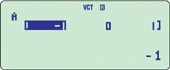# CASIO FX 991 ES PLUS SCIENTIFIC CALCULATOR

100.00

The FX991 ES PLUS has many functions that can be use to solve many problems. Our users say they love the fact that the scientific calculator use two types of power, one solar and the other battery so is ECO friendly and you are safe to take it into an exam without it failing not to work. Others opinions of the product is that they think that it is one to the best calculator that you can buy. One of the advantages that we are told about is that the result that you get you able to use the same figure in the next part of the sum, without having to round up numbers or to re-type up the whole digits which can lead to errors. Other great functions that we are told about was that you are able to use the calucator to work back wards, such as in algebraic equations. This can make life so much easier or so our users tell us.

The high technical specifications make this the most powereful Scientific at this price point.You can use this calculator from School to University and on into work.

# fx-991ES PLUS• The colors may differ slightly from the original.

## fx-991ES PLUS

Number of Functions : 417
Natural textbook display
Solar & Battery

## Features

### New functions

• New equation mode
• Random integers

### Standard functions

• Fraction calculations
• Combination and permutation
• Statistics (List-based STAT data editor, standard deviation, regression analysis)
• 9 variables
• Table function
• Comes with new slide-on hard case

### fx-82ES PLUS/85ES PLUS/350ES PLUS functions, in addition to:

• Equation calculations
• Integration/differential calculations
• Matrix calculations
• Vector calculations
• Complex number calculations
• CALC function
• SOLVE function
• Base-n calculation

#### Integration#### Complex number calculations#### Differential#### Vector#### Matrix operations## Specifications

• Non Programmable

•Number of Functions : 417

•Natural textbook displayDisplay expression same as textbook.

•10 + 2 digits10-digit mantissa + 2-digit exponential display.

•Dot matrix displayHigh-resolution screen provides beautiful looking graphs every time.

•Multi-replayQuick and easy recall of previously executed formulas for editing and re-execution.

•Solar & BatterySolar powered when light is sufficient, battery powered when light is insufficient.

•Plastic keysDesigned and engineered for easy operation.

• Basic Mathematical FunctionsTrigonometric, Exponential logarithmic, etc.

• Table GenerationYou can create a numerical table based on that expression by registering a function expression. In addition, graph functions can be represented by graphs.

•